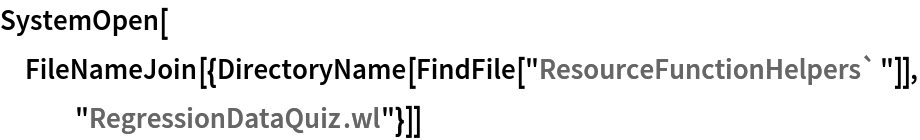Function Repository Resource:

Generate an interactive quiz on matching data to a quadratic regression curve

Contributed by: Nicholas E. Brunk, Wolfram|Alpha Math Team
 ResourceFunction["QuadraticRegressionDataQuiz"][] generates an interactive graphical quiz for users to specify points fitted by a requested quadratic curve.

## Details

ResourceFunction["QuadraticRegressionDataQuiz"] randomly chooses a quadratic curve of best fit. The user is then tested on their ability to generate a set of points whose nonlinear regression (as determined by NonlinearModelFit) matches the requested curve. Curve parameters of the best fit curve must be within 0.5 of the requested parameters in order to be marked as correct.
Click the graph to place a data point. Right-click a given data point to remove it from the list.
The interface returned by ResourceFunction["QuadraticRegressionDataQuiz"] includes the following buttons:
 Check answer automatically grades input with feedback Reset problem resets the problem by removing all chosen points Next problem randomly generates a new problem and internally saves the previous problem history Print report prints a description of the problems that have been attempted so far, all guesses made and whether the last guess on each problem was correct

## Examples

### Basic Examples (1)

Generate an interactive quiz on matching a specified quadratic regression curve:

 In:=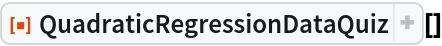Out=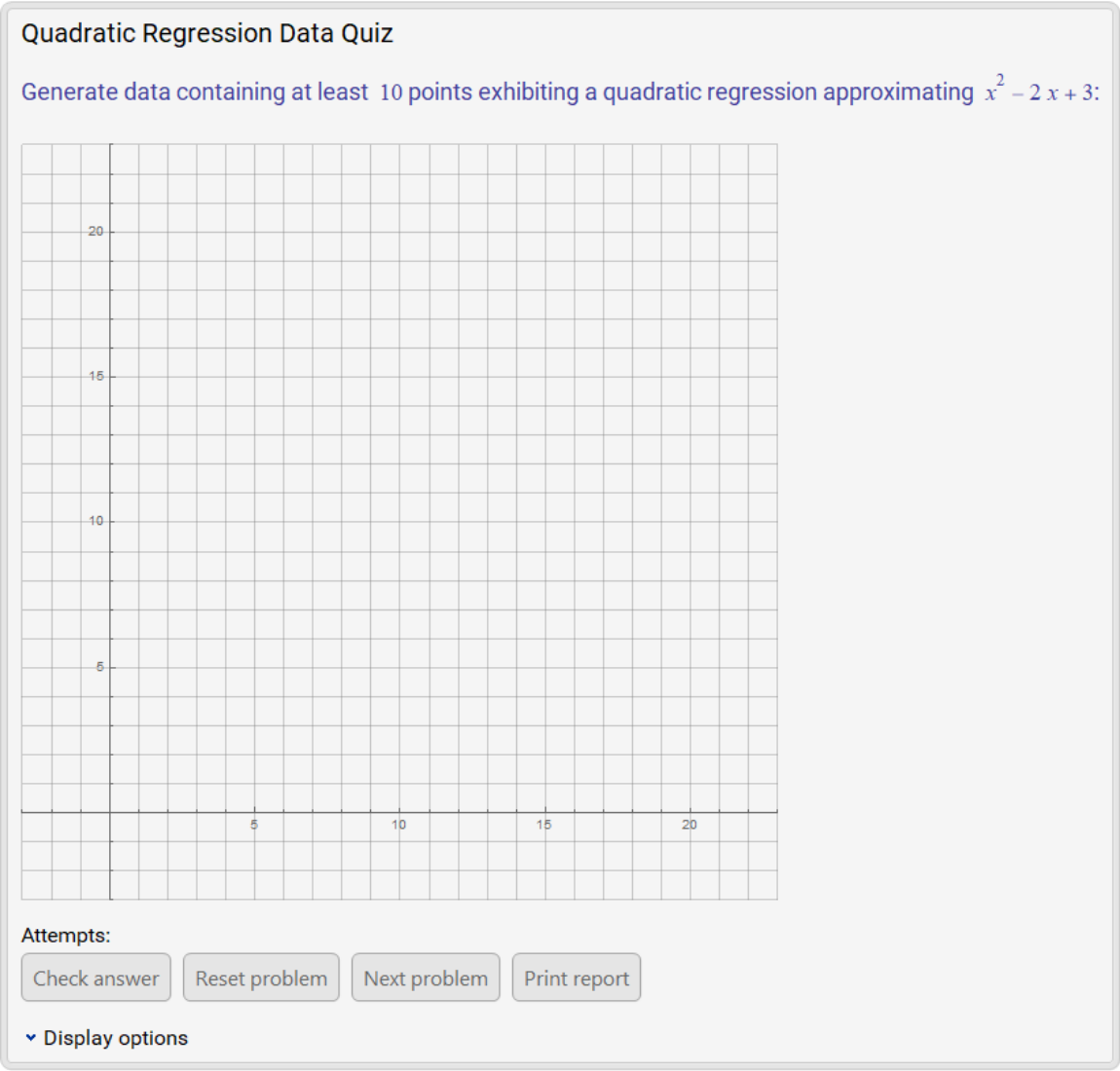### Scope (3)

Clicking Check answer marks an attempt based on the number of points and the approximate regression curve of the chosen points. Correct attempts are marked with a green check mark:

 In:=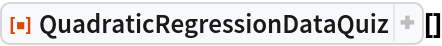Out=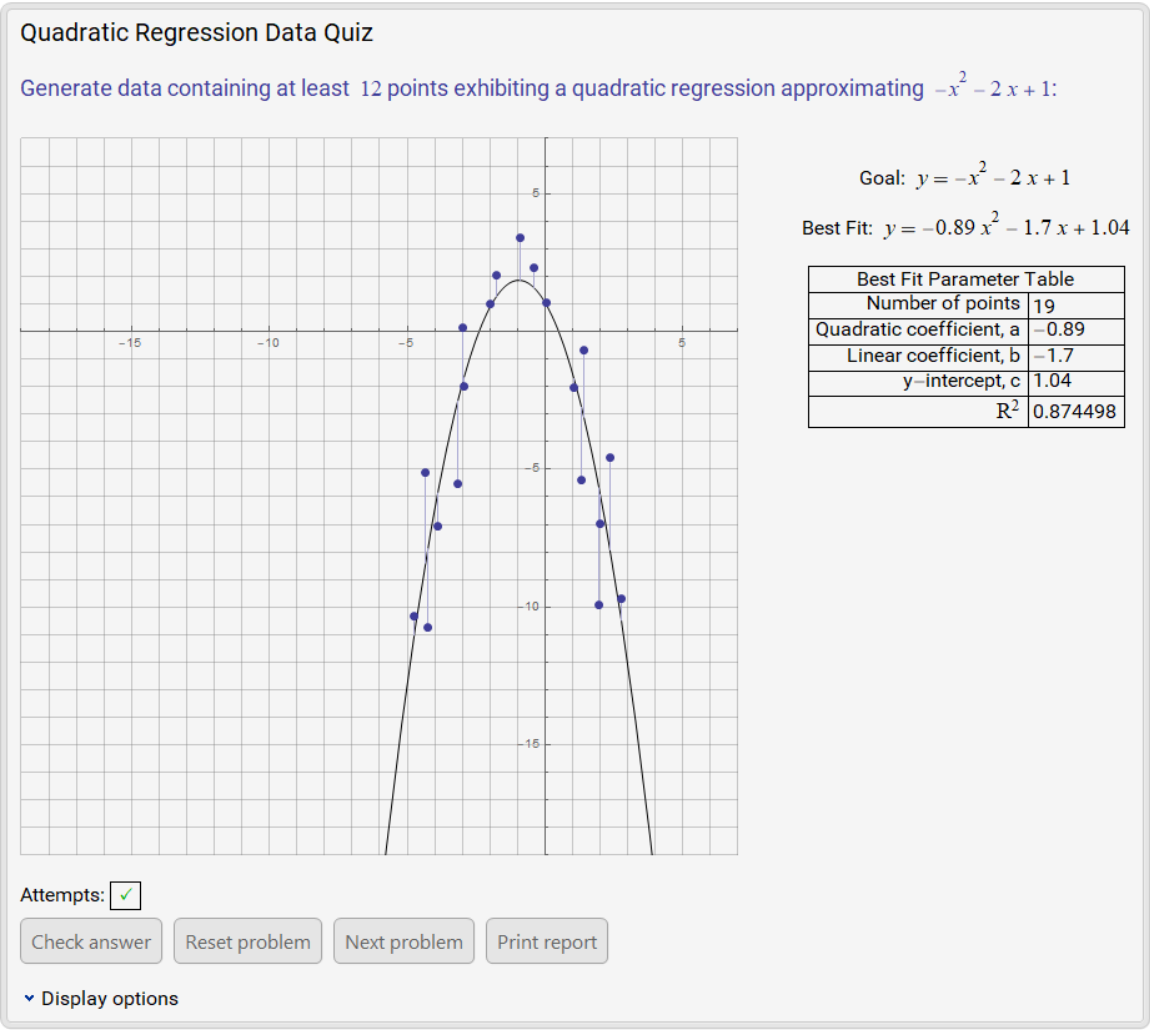If an attempt does not approximately meet the specified criteria, clicking Check answer marks the attempt as incorrect with a red x:

 In:=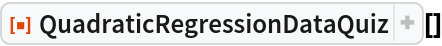Out=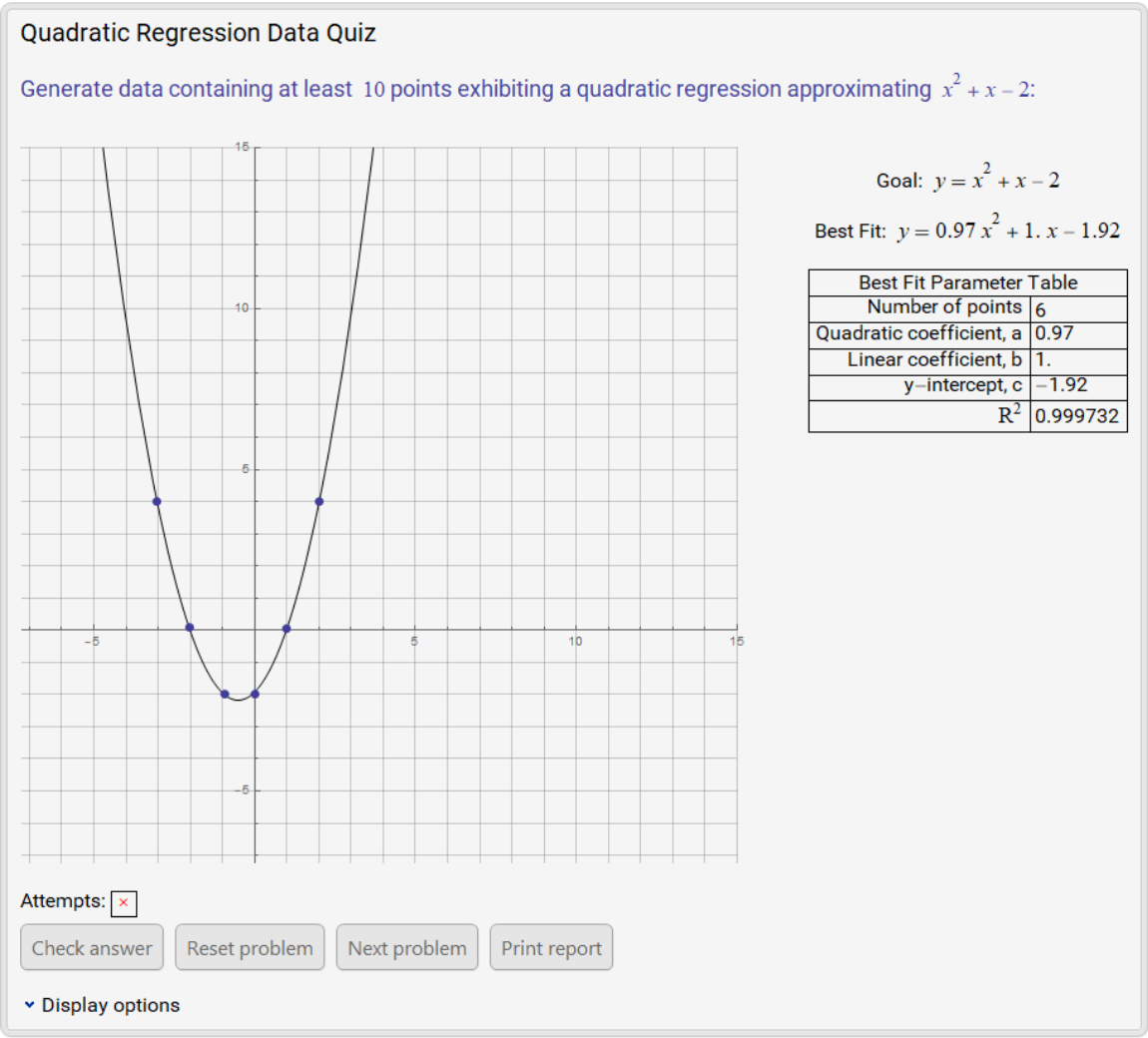Clicking the Print report button returns a description of the problems that have been attempted so far, all guesses made and if the last guess on each problem was correct:

 In:=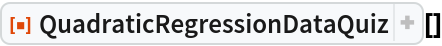Out=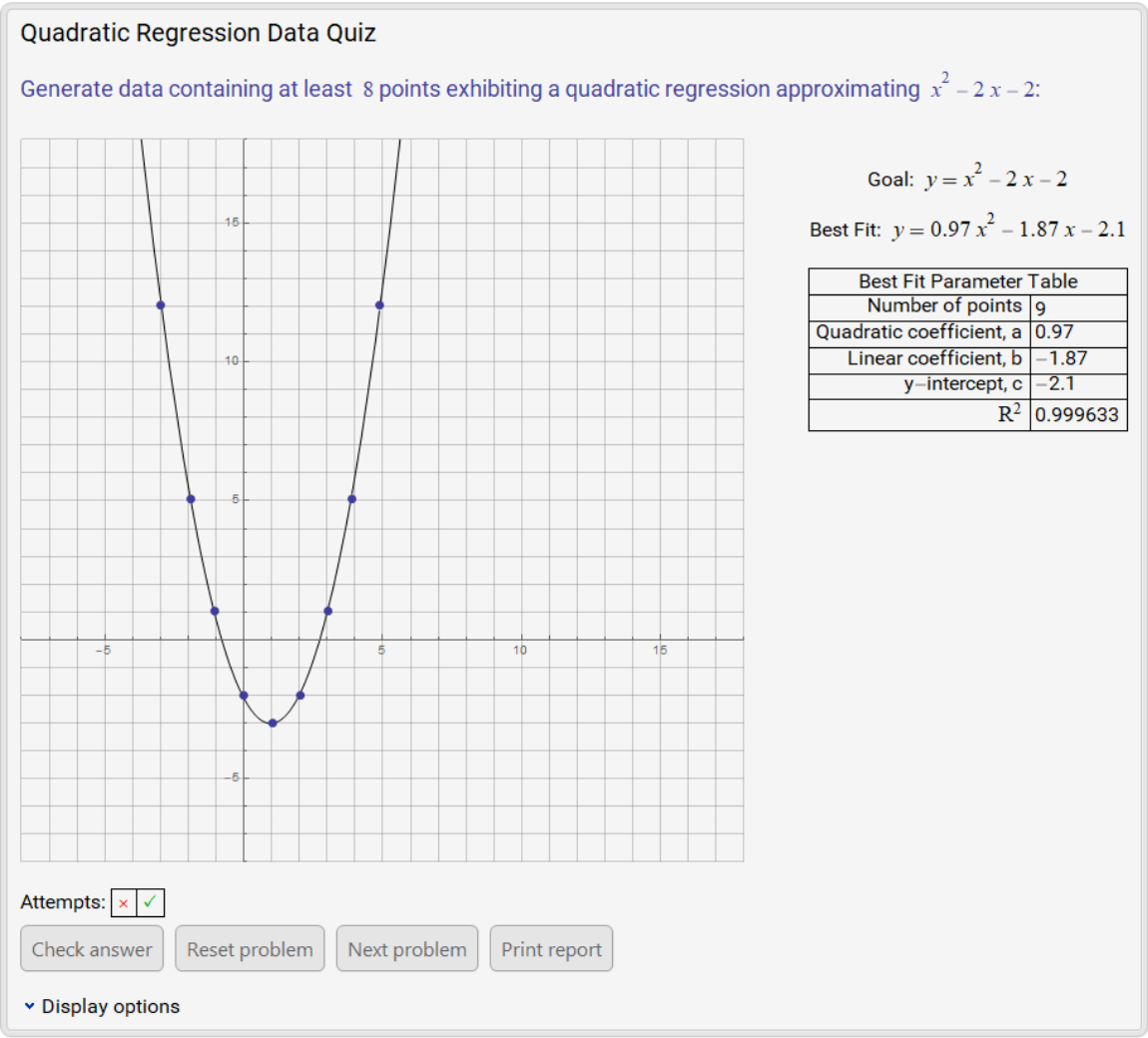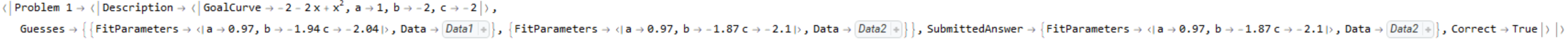## Publisher

Wolfram|Alpha Math Team

## Version History

• 2.0.0 – 23 March 2023
• 1.0.1 – 17 March 2021
• 1.0.0 – 22 February 2021

## Author Notes

To view the full source code for QuadraticRegressionDataQuiz, evaluate the following:

 In:=